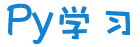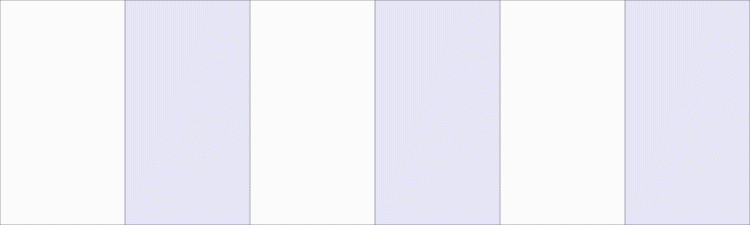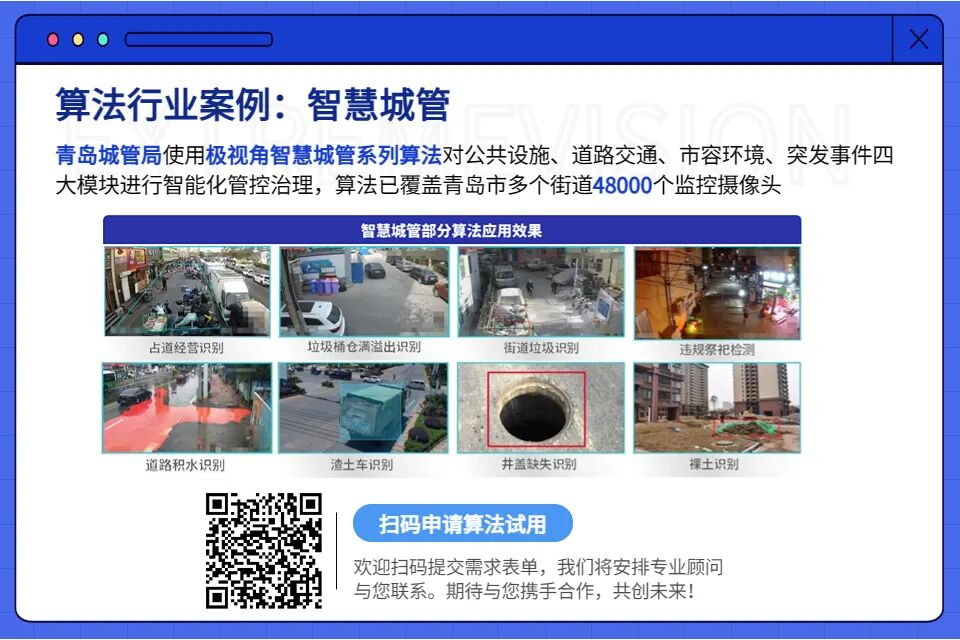注册    登录创作新主题

Python
 python开源   Django   Python   DjangoApp   pycharm
DATA
 docker   Elasticsearch

 问与答   闲聊   招聘   翻译   创业   分享发现   分享创造   求职   区块链   支付之战
aigc
 aigc   chatgpt
WEB开发
 linux   MongoDB   Redis   DATABASE   NGINX   其他Web框架   web工具   zookeeper   tornado   NoSql   Bootstrap   js   peewee   Git   bottle   IE   MQ   Jquery

Python88.com
 反馈   公告   社区推广

Py学习  »  Python

# 实践教程｜使用Python+OpenCV将照片变成卡通照片

↑ 点击蓝字 关注极市平台1. 加载图像
2. 创建边缘蒙版
3. 减少调色板
4. 结合边缘蒙版和彩色图像

``import cv2  import numpy as np  # required if you use Google Colab  from google.colab.patches import cv2\_imshow  from google.colab import files``

## 1. 加载图像

``def read\_file\(filename\):    img = cv2.imread\(filename\)    cv2\_imshow\(img\)    return img``

``uploaded = files.upload\(\)  filename = next\(iter\(uploaded\)\)  img = read\_file\(filename\)``

## 2. 创建边缘蒙版

``def edge\_mask\(img, line\_size, blur\_value\):    gray = cv2.cvtColor\(img, cv2.COLOR\_BGR2GRAY\)    gray\_blur = cv2.medianBlur\(gray, blur\_value\)    edges = cv2.adaptiveThreshold\(gray\_blur, 255, cv2.ADAPTIVE\_THRESH\_MEAN\_C, cv2.THRESH\_BINARY, line\_size, blur\_value\)    return edges ``

``line\_size = 7  blur\_value = 7  edges = edge\_mask\(img, line\_size, blur\_value\)  cv2\_imshow\(edges\)  ``

## 3. 减少调色板

### 色彩量化

``def color\_quantization\(img, k\):  # Transform the image    data = np.float32\(img\).reshape\(\(\-1, 3\)\)    # Determine criteria    criteria = \(cv2.TERM\_CRITERIA\_EPS + cv2.TERM\_CRITERIA\_MAX\_ITER, 20, 0.001\)    # Implementing K-Means    ret, label, center = cv2.kmeans\(data, k, None, criteria, 10, cv2.KMEANS\_RANDOM\_CENTERS\)    center = np.uint8\(center\)    result = center\[label.flatten\(\)\]    result = result.reshape\(img.shape\)    return result``

``total\_color = 9  img = color\_quantization\(img, total\_color\) ``

### 双边滤波器

``blurred = cv2.bilateralFilter\(img, d=7,   sigmaColor=200,sigmaSpace=200\) ``

• d：每个像素邻域的直径
• sigmaColor：参数值越大，表示半等色区域越大。
• sigmaSpace：参数的值越大，意味着更远的像素将相互影响，只要它们的颜色足够接近。

## 4. 结合边缘蒙版和彩色图像

``cartoon = cv2.bitwise\_and\(blurred, blurred, mask=edges\)``

1. https://www.programcreek.com/python/example/89394/cv2.kmeans
2. http://datahacker.rs/002-opencv-projects-how-to-cartoonize-an-image-with-opencv-in-python/Python社区是高质量的Python/Django开发社区